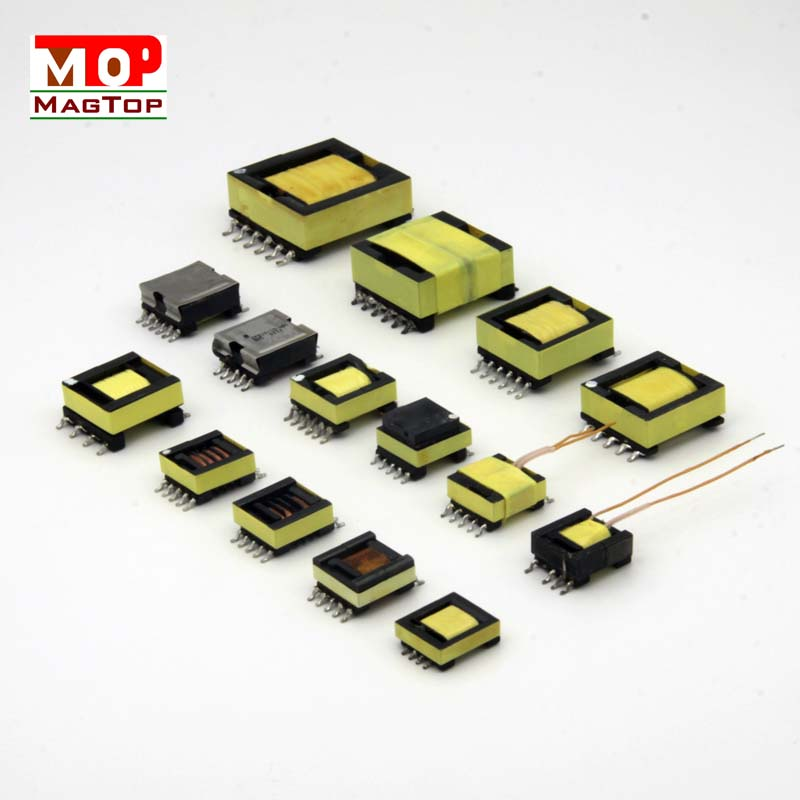# Simple design of power transformer

##### author: MagTop
01/02/2023Company News | common mode chokes | Industry News | transformer|RJ45 connectors

Power transformers are low-frequency transformers.
The method introduced in this paper is suitable for the design of common AC transformer below 50Hz 1 kW.
(1) The core of the power transformer.
It is usually made of silicon steel sheet.
The thinner the silicon steel sheet, the smaller the power loss, the better the effect.
The whole core is made up of many silicon steel sheets, each of which is insulated.
The purchased silicon steel sheet has a layer of non-conductive oxide film on its surface, which has sufficient insulation capacity.
See the following article for the common standard core sheet specifications of domestic small power transformers.
(2) Simple design of power transformer.
Design a power transformer, mainly according to the power to choose the transformer core cross-sectional area, calculate the number of the primary stage of each coil, etc.
The so-called core cross-sectional area S is the product of the standard size a of the middle tongue of the silicon steel sheet and the total thickness b stacked up.
If the primary voltage of the power transformer is U1, secondary n groups, each group of voltage is U21,U22,┅,U2n, each group of current is I21,I22,┅,I2n...
The calculation steps are as follows:
First step, calculate the secondary power P2. Secondary power is equal to the sum of the secondary power, that is, P2 =U21*I21+U22*I22+┅+U2n*I2n.
Step 2: Calculate the power of the transformer P.
Considering the efficiency of the transformer is η, then the primary power P1=P2/η,η is generally between 0.8 ~ 0.9.
The power of the transformer is half the sum of the primary and secondary power, which is P=(P1+P2)/2
The third step is to check the core cross-sectional area S. According to the transformer power, calculate the core cross-sectional area S by Formula (2.1), and select the core sheet specification and stack thickness from the standard core sheet specification table commonly used for domestic small power transformers.
Step 4: Determine the winding number per volt N. According to the cross-sectional area S of the core and the magnetic flux density B of the core, the winding number per volt of the primary coil N is obtained from Equation (2.2).
The B value of the core can be selected as follows: for the silicon steel sheet of good quality, take 11000 gauss;
General silicon steel sheet, take 10000 gauss;
Iron sheet, 7,000 gauss.
As for the voltage drop of the wire resistance, the number of turns per volt of the secondary coil N' should be increased by 5% ~ 10% compared with N, that is, N' should be selected between 1.05N ~ 1.1N.
The fifth step is the calculation of the primary coil.
Primary coil N1 = N * U1. Secondary coil N21 = N '* U21, N22 = N * U22 ┅, N2 = N' * U2n.
Step six, check the diameter of the wire.
According to the current size of each coil and the selected current density, the wire diameter of each coil can be obtained from Equation (2.3).
General power supply transformer current density can choose 3 A/mm?
Step 7: Check.
According to the calculation results, calculate the number of coils and layers of each layer, and then calculate the size of the coil, to see whether the window can be put down.
If not, you can increase one core, if space, you can reduce one core.
Using the national standard GEI core, and tongue width a and stack thickness b ratio between 1:1 ~ 1:1.7, coil is put down.
The calculation formula of each parameter is as follows:
ln(S)=0.498*ln(P)+0.22 ┅(2.1)
Ln (N) = ln (P) - 0.494 * 0.317 * ln (B) + 6.439 ┅ (2.2)
Ln (D) = 0.503 * ln (I) to 0.221 ┅ (2.3)

Variable specification
P: Power of the transformer.
Unit: Watt (W)
B: Working magnetic flux density of silicon steel sheet.
Unit: Gauss (Gs)
S: Cross-sectional area of the core.
Unit: cm2
N: number of coils per volt.
Unit: cycle per volt (N/V)
I: Use current.
Unit: Ann (A)
D: conductor diameter.
Unit: mm (mm)